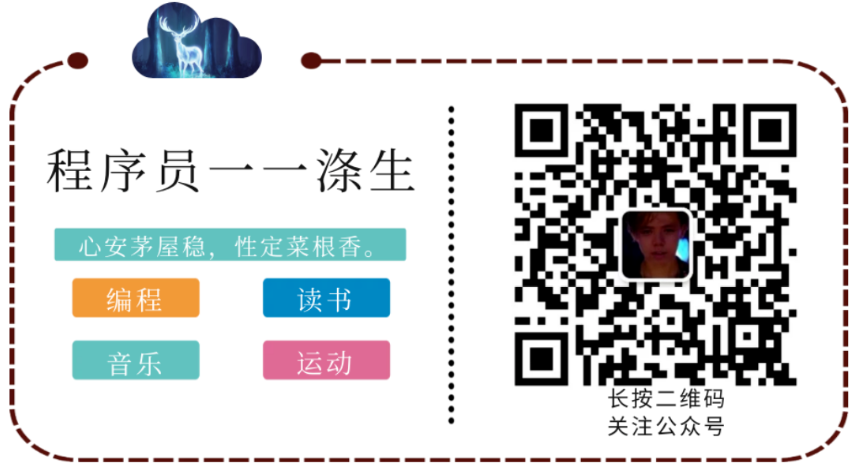Categories

This article try to stick together BERT of the original paper, but considering the easy to understand, so not sentence by sentence translation, but the translation according to the author’s personal understanding, some papers did not explain clearly the author failed to place or deep understanding, there original release, if inappropriate, please include a lot and want to be guided and corrected.

Posted

Bert: Bidirectional Encoder Representations from Transformers
A method of characterizing a model from the model Transformers come bidirectionally encoded.

• https://arxiv.org/pdf/1810.04805

### Abstract

BERT design is expressed by the left and right bidirectional context jointly adjusted in all layers, deep trained beforehand from unlabelled text.

BERT pre-trained model can be created by fine-tuned in a wide range of tasks in the new best record, such as Q & A tasks, verbal reasoning tasks, without having to make substantial changes to the architecture itself BERT.

### 1 Introduction

BERT is a simple concept, the result of strong practice model. It created a new best record in 11 natural language processing tasks.

ELMo is based on feature-based methods [Note 2] Application of the pre-trained language representations.

OpenAI GPT method is based on fine-tuning [Note 3] Application of the pre-trained language representations.

The above two methods in the training phase, we share the same objective function, they use single language model to learn common language representation.

For authors believe that the sentence, the way attention is suboptimal, for token-level tasks, such as Q & A tasks, it will bring a bad effect. Because a similar question and answer tasks, based on a combination of the two directions context is very important.

In this thesis, the author proposes BERT model to improve the method based on fine-tuning.
BERT: Bidirectional Encoder Representations from Transformers.
BERT inspired cloze task by using a “masked language model” (MLM) pre-training goals to reduce one-way constraints mentioned above.
MLM randomly masks out some of the input tokens, their goal is predicted in the original vocabulary id from the context of these tokens. Pre-training language model does not want left-to-right’s, MLM target characterization makes the integration of contextual information left and right, which allows the author to a depth of two-way pre-training Transformer models. In addition to MLM, the authors also used a “next sentence prediction” task, the associated text-pair characterization of pre-training. The contribution of this paper is as follows:

• Demonstrating the importance of two-way pre-trained language representation. BERT MLM using such models may be pre-depth characterization bidirectional training; the GPT using unidirectional pre-trained on a language model; ELMO using good left-to-right and right-to-left, respectively, characterized by the training, and then only with a simple series .

• Shows the characterization of pre-training can reduce the need for many engineering onerous task-specific architecture. BERT is the first to achieve the best performance on a huge order of sentence and word level task-based representation model of fine-tuning.

• BERT best record breaking 11 NLP tasks. Code and pre-training model can be obtained from here.

### 2 Related Work

Pre common language to characterize training has been quite a long history. This section provides a brief look at the use of the most widely used general language to characterize pre-trained.

#### 2.1 Unsupervised Feature-based Approaches

For decades, learning widely used word representation has been an active area of ​​research, the field of neural and non-neural area include. Pre-term training is a major part of modern NLP embedded systems, providing scratch learning words embedded in a significant improvement. In order to embed pre-term training vectors, people used the left-to-right language modeling goals, as well as to distinguish correct and incorrect modeling target words from the left and right context.
These methods have been extended to a more coarse particle size, such as embedded in sentences, paragraphs, or embedded. In order to characterize the training sentence, previous work has used these objectives: ranking the candidate sentences; Characterization of a given sentence on, left-to-right next sentence generation; automatic denoising from the encoder.

ELMo and its predecessor summarizes the research of traditional embedded word from a different dimension. Context sensitive feature extraction thereof from the left-to-right and right-to-left language model. Context characterizing each token (word, symbol or the like) is performed by left-to-right and characterization of the right-to-left series obtained. After the word in context embedded architecture and has a specific task, ELMo NLP in several key benchmarks (including: questions and answers, sentiment analysis, named entity recognition) achieved the best record. Melamud, who in 2016 proposed the use of LSTMs model to learn about the context of a context characterized by the words of a prediction task. And ELMo similar, their model is based on feature-based methods, and there is no depth two-way (Note 1). Fedus, who in 2018 demonstrated the cloze task can be used to improve the robustness of the text generation model.

### 2.2 Unsupervised Fine-tuning Approaches

As with the feature-based method, the pre-training Fangxiang Gang started just words on unmarked text embedded parameters (unsupervised learning).
Recently, other documents and generating sentence context token encoder characterized unlabeled text already pre-trained, and by fine-tuned manner as the downstream task. The advantage of these methods is that very few parameters need to learn from scratch. At least in part because of this advantage, before OpenAI GPT on a number of sentence-level mission from GLUE benchmark reached an optimum level. Left-to-right language modeling and automatic encoder target for this training model.

### 2.3 Transfer Learning from Supervised Data

There is also work to do to show the effectiveness of the transfer learning from a large data set of supervisory tasks, like natural language reasoning (NLI), and machine translation. Computer vision research also demonstrates the importance of migration learning, an effective technique is fine-tuning (fine-tune) ImageNet of pre-training model.

### 3 BERT

This section describes the detailed implementation of BERT. Using BERT has two steps: pre-training and fine-tuning. During the pre-training, BERT model is trained on the different tasks of unlabeled data. When trimming, BERT model pre-trained is initialized with parameters, and is based on the label data to a downstream task training. Each task has its own fine-tuning downstream model, despite initial pre-training all the time with good BERT model parameters. In FIG 1, an example of the present art section Q a sample run.

Figure 1: pre-training process and the operation of fine-tuning BERT. In addition to output layer, the two-stage architecture is the same. Pre-training model parameter initialization parameters of the model will be as different downstream tasks. When fine-tuning, all the parameters involved in fine-tuning. Symbol [the CLS] When a particular set is added in front of each input sample, indicates that this is a start of input samples, [the SEP] is a special set of division marks. Such partition questions / answers.

BERT a distinctive characteristic is its unified architecture across tasks, namely the smallest difference between the pre-training infrastructure and downstream infrastructure.

#### Model Architecture

BERT model architecture is a multi-layered two-way Transformer encoder (about Transformer can see this article). Because Transformer use became widespread, and BERT associated with the Transformer and the original Tranformer achieve almost the same, so this paper will not elaborate, I recommend the reader to see the original Transformer papers, as well as “The Annotated Transformer” (This is the original an excellent explanation of the thesis set forth Transformer).

Here, L denotes the number of layers of indicating, H represents the number of dimensions of each of the hidden units, A represents the number of self-attention header. BERT There are two kinds of model size, namely BERT (base, L = 12, H = 768, A = 12, Total Parameters = 110M) and BERT (large, L = 24, H = 1024, A = 16, Total Parameters = 340M).

Same BERT (base) and a set of model size OpenAI GPT, to facilitate comparison. It is important to note is, BERT Transformer bidirectional self-attention, while GPT
Transformer using self-attention constrained, each token can only note that the context in which it left.

#### Input/Output Representations

BERT made using various downstream task, a token in the input representation may clearly in sequence a sentence or a pair of sentences (such as ). Here the “sentence” does not have to be language sentence, but can be any range of continuous text. “Sequence” refers to a BERT input sequence, and may be a sentence, it may be packaged together two sentences.

The authors used the word to do WordPiece embeddings embedded, corresponding vocabulary has 30,000 token. The first token of each sequence is always a particular classification token ([CLS]). This last token corresponding to the hidden state sequence is characterized as a polymerization classification task. Packaged into a sequence of sentences. There are two methods for distinguishing the sentence of the sentence. First, by delimiter [the SEP]; second, a model architecture added after learning embedded (learned embedding) to each token, to indicate that it is part of a sentence or a sentence A B. As shown in FIG. 1, E represents an input word embedded, C represents the vector of the last hidden layer [the CLS] is, Ti denotes the i th input vector in the last token of the hidden layer.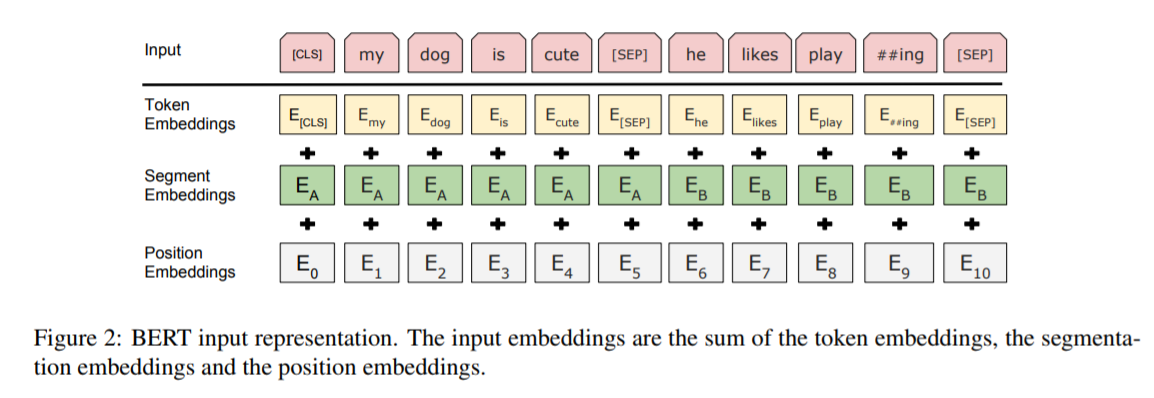#### 3.1 Pre-training BERT

Intuitively, the authors reason to believe that a depth of two-way model will indeed be stronger than the one-way or two-way shallow model.
Unfortunately, the standard conditions in accordance with the language model only from left-to-right or right-to-left way of training, until conditions permit two-way each word indirect “see itself”, and can predict the target in the context of a multi-layer word.
Unfortunately, standard conditional language models can only be trained left-to-right or right-to-left, since bidirectional conditioning would allow each word to indirectly “see itself”, and the model could trivially predict the target word in a multi-layered context. (original sentence)

In order to characterize a two-way depth training, some of the percentage of simple random mask input tokens, those tokens are then predicted mask off. This step is called “masked LM” (MLM), although it is often called cloze tasks (Cloze task) in the literature.
tokens corresponding to mask out the last hidden layer feed a vector output softmax, as in the standard as in LM. In the experiment, the authors lost 15% of WordPiece tokens for each sequence of random mask. And denoising auto-encoders compared, BERT practice is predicted to be only mask out the word, rather than a complete reconstruction of the input.

Although this allows the author to obtain the two-way pre-training model, its negative impact is between the pre-training and fine-tune the model to create a mismatch, because [MASK] symbol will not appear in the fine-tuning stage. So, we need a way to get those words out of the mask is also characterized by the original model to learn, so here the authors used a number of strategies, specifically refer to: Appendix A.1.

##### Task 2:Next Sentence Prediction (NSP)

Many downstream tasks, such as questions and answers, natural language reasoning, need-based understanding of the relationship between the two sentences, and this relationship can not be obtained by direct modeling language to. In order to train a possible model for understanding the relationship between sentences, the authors forecast for the next sentence a binary classification task was pre-trained, these sentences can be obtained from any of the corpus into a single language. In particular, when a sentence is selected for each of the prediction samples A and B, 50% of the time B is behind the next sentence A (labeled IsNext), 50% of the time B is a random sentence corpus (labeled as NotNext). In FIG. 1, C is used to predict the next sentence (NSP). Although simple, this method NLI and QA tasks are very helpful. Section 5.1 of this have to show.

NSP tasks and Jernite et al. Is closely related to learning objectives expressed in (2018) (2017) and Logeswaran and Lee. Task, previous work, the sentence is embedded only transferred to the downstream task, BERT and transfer all parameters of the terminal to initialize the parameters of the task model.

Pre-training data with reference to the pre-training process is largely pre-existing language model training literature. Pre aspects of training data, the authors used BooksCorpus (800M words), English Wikipedia (2500M words). Wikipedia authors to extract only the text of the paragraph, ignore lists, tables and titles. In order to extract long continuous sequence, the key is to use the document corpus level, rather than disordered Sentence corpus like the one billion word benchmark (Chelba et al., 2013).

#### 3.2 Fine-tuning BERT

Trimming is very simple, because the self-attention mechanism allows Transformer BERT by exchanging the appropriate input and output to a downstream task for many modeling – whether single text or text pair. For applications involving common pattern of the text is distinguished on the encoded text in the text, then apply two-way cross attention. BERT using unified mechanism of self-attention these two steps, the use of self-attention BERT encoding a series of text pairs, which contains the process attention way crossover between the two sentences.
Input sentence A and the sentence B may be: (1) the interpretation of the sentence (2) the assumption of a sentence (3) Q sentences of text-∅ to (4) or text categorization sequence dimensions.
An output terminal, for, characterizing feed a token for token level output layer task sequence labeling and Q are similar, [the CLS] Characterization feed a classifier output layer, such as emotional analysis.

Trimming the cost of much smaller than the pre-training. Many of the paper the results are exactly the same from a pre-training model began in the TPU only takes one hour can reproduce, the GPU is also just a few hours. More details can be viewed in Appendix A.5

### 4 Experiments

This section shows BERT fine-tuning the results on 11 NLP tasks.

#### 4.1 GLUE （General Lanuage Understanding Evaluation）

GLUE benchmark is a series of different natural language understanding tasks. GLUE detailed description of the data set in the appendix B.1.

fine-tune the GLUE, Section 3 describes the use of sentences and sentence-pairs with the final vector C as characterized Hide, C corresponding to the first input token ([CLS]). Categorizing layer weight coefficient matrix W (shape: K × H), K is the number of categories. The author uses the C and W computing standard classification of losses, such as log (softmax (C · W)).

GLUE on all tasks, the authors used a batch-size = 32, epochs = 3. For each task, you have to choose the best learning rate by fine-tuning the development of validation set (in 5e- 5,4e – between 5,3e -5 and 2e-5). In addition, BERT for large models, the authors found that fine-tuning sometimes unstable in a small data set, so random reboots a few times, and choose the best performance on a set of model development. With random restarts, we use the same pre-trained checkpoint but perform different fine-tuning data shuffling and classifier layer initialization.9 (?)

BERT base version of the model architecture and OpenAI GPUT in addition to attention masking outside, almost the same.
BERT large version significantly higher than the base version to perform better. About the size of the impact model, the 5.2 has more in-depth discussion.

This is a quiz on the collection of 100k. Given a problem and an essay, and the corresponding answer, the task is to predict the answer text span (the answer text span in the passage) in the essay.
As shown in FIG. 1, in the task Q, the input of the problems and represented as a sequence of short, which represents a problem to use embedded A, B represents a short embedded. When fine-tuning, the authors introduce a start vector S, and an end vector E, dimensions are H. Starting word answer span of word i probability calculation formula:

The answer at the end of the word indicates the probability of the same principle.
Fraction is defined position i to position j candidate span as follows:

And will meet j> i maximum score of span most predictions. Training goal is the correct number of start and end positions and the likelihood estimation.
Fine-tuning of the three epochs, the learning rate is set to 5e-5, batch-size is set to 32.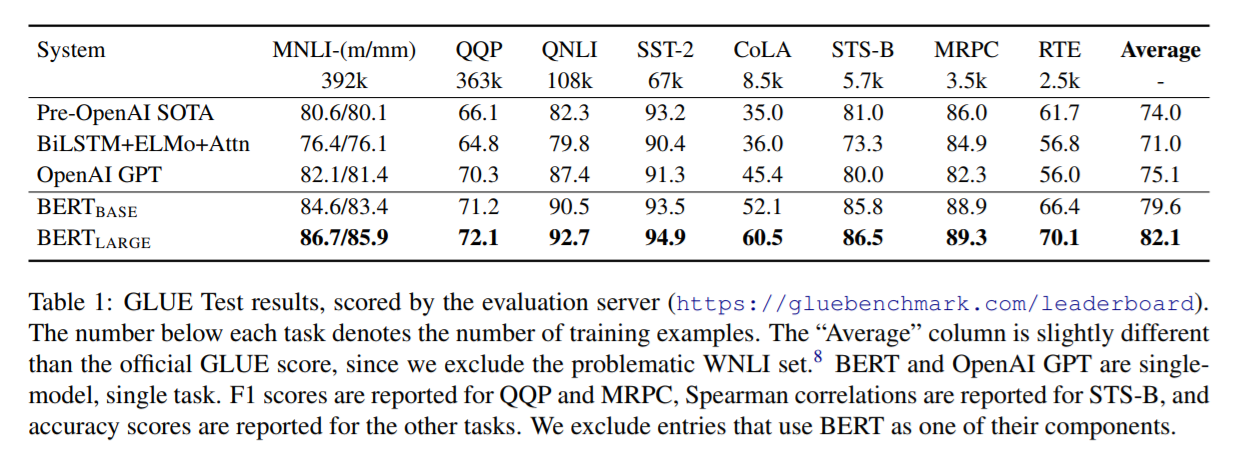Table2 shows the top-ranking weed and results. Wherein the weed in the ranking described SQuAD not have the latest common system, and allows the use of any public data training respective network.
Thus, use of appropriate data in the system enhancement, firstly TriviaQA fine-tune (Joshi et al., 2017), and then fine-tuning of SQuAD.

We treat questions that do not have an answer as having an answer span with start and end at the [CLS] token. The probability space for the start and end answer span positions is extended to include the position of the [CLS] token. For prediction, we compare the score of the no-answer span: snull = S·C + E·C to the score of the best non-null span

sˆi,j = maxj≥i S·Ti + E·Tj . We predict a non-null answer when sˆi,j > snull + τ , where the threshold τ is selected on the dev set to maximize F1. We did not use TriviaQA data for this model. We fine-tuned for 2 epochs with a learning rate of 5e-5 and a batch size of 48.

#### 4.4 SWAG

The Situations With Adversarial Generations (SWAG) data set contains knowledge-based reasoning 113k complete sentence for example, for evaluation. Given a sentence, the task is to choose from four options most likely is the continuation (continuation / extension).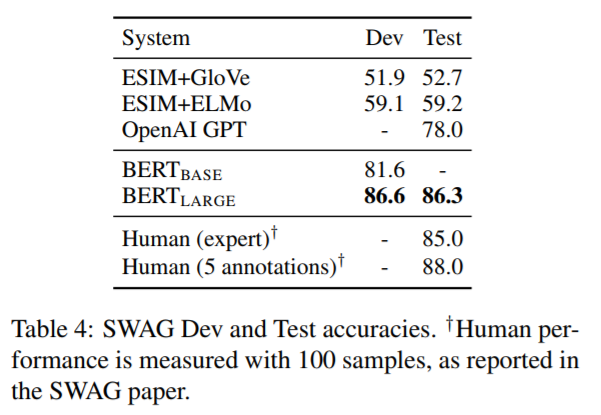5 Ablation Studies ablation studies

This section by BERT in all aspects of doing ablation experiments to understand the relative importance of the part.

#### 5.1 Effect of Pre-training Tasks

After removing by NSP, characterization and comparison of two-way BERT Left-to-Right characterization, the authors have proved that there NSP better and more effective two-way characterization.
By introducing a two-way LSTM, authors demonstrated that BILSTM can get better results than the Left-to-Right, but still not a good base version of BERT effect.
Comparative results are shown in particular:

In addition, with regard to training ELMo respectively as RTL and LTR way, the author also gives the place its not as BERT:

• this is twice as expensive as a single bidirectional model;
• this is non-intuitive for tasks like QA, since the RTL model would not be able to condition the answer on the question;
• this it is strictly less powerful than a deep bidirectional model, since it can use both left and right context at every layer.

#### 5.2 Effect of Model Size

Table6展示了对比结果。大模型带来更好的表现。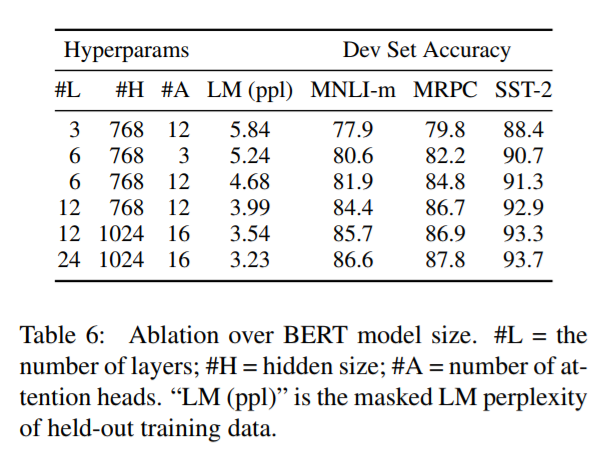For example,
the largest Transformer explored in Vaswani et al. (2017) is (L=6, H=1024, A=16) with 100M parameters for the encoder, the largest Transformer we have found in the literature is (L=64, H=512, A=2) with 235M parameters (Al-Rfou et al., 2018). By contrast,
BERT(base) contains 110M parameters
BERT(large) contains 340M parameters.

Finally, the conclusions of this section are as follows:
we hypothesize that when the model is fine-tuned directly on the downstream tasks and uses only a very small number of randomly initialized additional parameters, the taskspecific models can benefit from the larger, more expressive pre-trained representations even when downstream task data is very small.
Roughly meaning, by fine-tuning downstream task even if the amount of data that can provide very small, you can still take advantage of pre-training model to get good training effect.

#### 5.3 Feature-based Approach with BERT

Compared to the above it has been said of fine-tuning the way, feature-based way also has its key advantage.
First, not all tasks can be easily expressed as Trasformer encoder architecture, so there will need to add a demand model architecture based on a specific task.
Second, pre-calculated represents a costly training data, and then express on the use of cheaper models run many experiments, which is of great benefit to the calculation.

In this section, the authors compared the fine-tuning and feature-based way in the NER application of BERT.
BERT’s input, using the case of a reserved word model, and contains the maximum document context data provided. According to standard practice, the author expressed as mark tasks, but without the use of CRF in the output layer. Use of a first sub-token characterization, as an input token-level classifier of NER.

In order to do and fine-tuning method ablation experiments, the author no way to extract activations from any fine-tune the parameters of one or more applications feature-based methods. Embedded in these contexts to make use of a random initialization BiLSTM two-dimensional input 768, and then fed to the classifier layer.

Table 7 shows the results:

See, feature-based methods, the last four stitching hidden layer manner, fraction F1 may reach 96.1 and only less than 0.3 BERT (base).
The results showed that, BERT two kinds of application methods are valid.

### 6 Conclusion

Recently example by transfer learning to improve learning model suggests a rich, unsupervised pre-training is an important component of many language understanding systems. In particular, these results make even low-resource tasks can also benefit from the deep-way architecture.
The main contribution BERT is further extended to those found deep bi-directional architecture, so that the same model can be successfully pre-trained to deal with a broad set of NLP tasks.

Appendix A Additional Details for BERT

#### A.1 Illustration of the Pre-training Tasks

Here the author provides a sample of pre-trained.

Masked LM and the Masking Procedure assumption that the original sentence was “my dog ​​is hairy”, the authors mentioned in 3.1 Task1 will be randomly selected 15% of the sentences were tokens position mask, randomly selected here to assume the position for the fourth token the mask off, which is carried out for hairy mask, then the mask process can be described as follows:

80% of the time: Replace with the target word [MASK], for example: my dog ​​is hairy -> my dog ​​is [MASK].

10% of the time: the word is replaced with a random target word, for example: my dog ​​is hairy -> my dog ​​is apple.

10% of the time: do not change the target word, for example: my dog ​​is hairy -> my dog ​​is hairy. (The purpose of this is to tend to characterize the observed actual word.)

The above process, combined with the need to understand the process of training epochs, each epoch finished school again represents all the samples, each sample in multiple epochs process is repeated input into the model, knowing this concept, above 80%, 10%, 10% like to understand, that when a sample feeding each model, the probability of replacement target word with [MASK] is 80%; the probability of replacement target word in a random word 10%; the target word does not change the probability is 10%.

BERT introduce some articles to explain the MLM process when 80% here, 10%, 10% construed to replace the original sentences were randomly selected 15% of the tokens in the replacement of 80% of the target word with [MASK], 10% replacement target word random words, 10% of the target word does not change. This understanding is wrong.

Then, the author talked about the benefits of taking the above mask strategy in the paper. Bottom line is that after using the above strategy, Transformer encoder does not know which word will make its forecast, or did not know which word will be randomly word to replace, then it had to characterize a context for each input token remains distribution (a distributional contextual representation). This means that if the model you want to learn to predict what the words are, it will lose the learning context information, and if the model training process can not learn to predict which word is, then it must be judged by the learning context of the information We need to predict the word, such a model that has the ability to express the characteristic sentence. Further, since the probability of occurrence of random replacement of all tokens relative sentence only 1.5% (i.e., 15% of 10%), and therefore will not affect the language understanding model. In this regard, C.2 section of this paper made to assess the impact of this process.

Compared to standard language model training, masked LM tokens in each batch in only 15% of the portions are predicted, the model convergence requires more pre-training step. C.1 section demonstrates slower MLM (predict will each token) than left-to-right model of convergence, but to improve learning outcomes far more than the increase in training costs.

Next Sentence Prediction
“The next sentence prediction,” the task examples:

``````Input = [CLS] the man went to [MASK] store [SEP]
he bought a gallon [MASK] milk [SEP]

Label = IsNext

Input = [CLS] the man [MASK] to the store [SEP]
penguin [MASK] are flight ##less birds [SEP]

Label = NotNext``````

A.2 Pre-training Procedure pre-training process

This section starts with a sample under a prediction task acquisition strategy is to select two span roughly from the text corpus, span here can be understood as a complete word. Then, two corresponding sentence span sentences A and B. Wherein 50% of the cases, the sentence is a sentence B is an A, whereas 50% of the cases, lower than A, a B. And, the sentence A and B are combined to a length <= 16="" 40="" 64="" 128="" 256="" 512="" tokens.=""     Then="" introduced="" the="" segmentation="" of="" LM="" cases:=""     The="" masking="" is="" applied="" after="" WordPiece="" tokenization="" with="" a="" uniform="" rate="" 15%,="" and="" no="" special="" consideration="" given="" to="" partial="" word="" pieces.?=""     On="" pre-training="" time="" using="" batch-size="256," which="" means="" that="" each="" batch="" consists="" *="" tokens,="" trained="" total="" 1,000,000="" steps,="" nearly="" epochs,="" more="" than="" 3.3="" billion="" words.="" Adam="" gradient="" optimization="" algorithm,="" learning="" β1="0.9," L2="" right="" β2="0.999,0.01" heavy="" attenuation="" warmup="" in="" first="" step="" ="" Note="" 4,="" followed="" by="" linear="" attenuation.="" The="" authors="" used="" 0.1="" probability="" all="" layers="" dropout.="" On="" activation="" function,="" chose="" gelu,="" instead="" standard="" relu,="" this="" choice="" OpenAI="" GPT.="" training="" loss="" sum="" mean="" masked="" likelihood="" next="" sentence="" prediction="" likelihood.="" (Training="" off="" language="" model="" predict="" lower="" sum.)=""     BERT="" base="" TPU="" cloud="" on="" four="" (a="" chips="" TPU).="" BERT="" large="" Each="" for="" days="" complete.=""     Since="" computational="" complexity="" square="" attention="" sequence="" length,="" so="" cost="" longer="" added="" expensive.="" To="" speed="" up="" process="" experiment,="" 90%="" 10%="" then="" remaining="" length="" pre-trained="" sequence,="" as="" learn="" embedded="" position="" (positional="" embeddings).<="" p=""/>

#### A.3 Fine-tuning Procedure

In the fine-tuning of the time, most super model parameters and pre-training time is the same, except batch-size, learning rate and epochs. The probability of dropout is always maintained at 0.1. Optimization value exceeds the parameter is specific to the task to do, but the author mentions the possible range of values ​​below, a good value within the range of tasks in the work of the cross:

• Batch size: 16, 32
• Learning rate (Adam): 5e-5, 3e-5, 2e-5
• Number of epochs: 2, 3, 4

The authors also observed 100,000 + training samples, ultra-parameter selection sensitivity is much lower than small data sets. Fine-tuning is still very fast, simple and crude runs on top of an exhaustive search to select a parameter that can make the best-performing model in the development of those parameters set manner also acceptable.

A.4 BERT, ELMo, OpenAI GPT contrast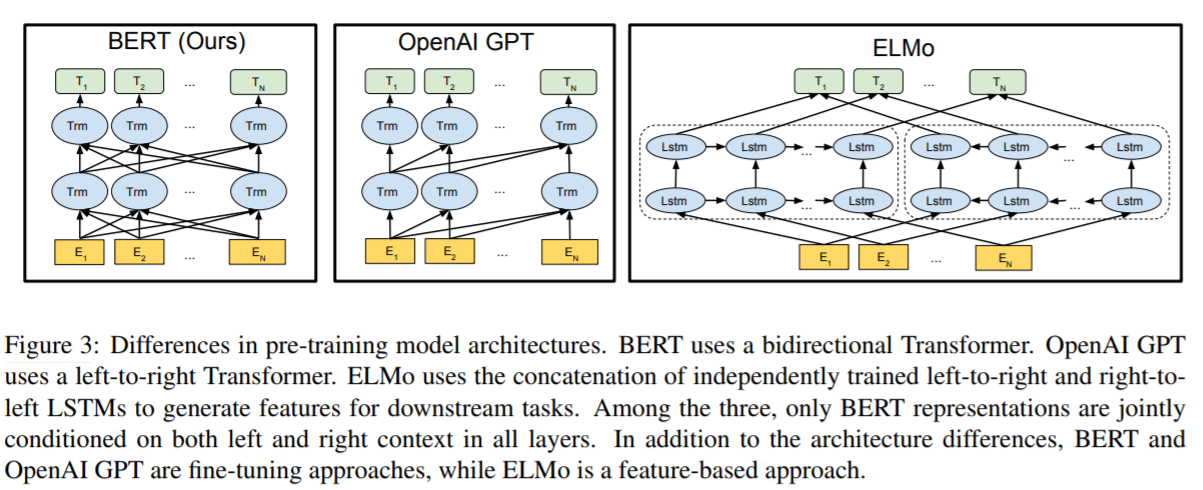BERT uses two-way Transformer architecture

OpenAI GPT using the Transformer left-to-right of

ELMo respectively using left-to-right and right-to-left independent training, and then outputs spliced ​​together, wherein the downstream sequence task to provide
The above three models architecture, characterized by BERT only model in each layer are jointly taken into account contextual information left and right.
In addition to different architectures, and further except that BERT OpenAI GPT is based on fine-tuning, and ELMo is based on the feature-based.

In addition to MLM and NSP, BERT and GPT in training when there are a few differences as follows:

• GPT is trained on the BooksCorpus (800M words); BERT is trained on the BooksCorpus (800M words) and Wikipedia (2,500M words).
• GPT uses a sentence separator ([SEP]) and classifier token ([CLS]) which are only introduced at fine-tuning time; BERT learns [SEP], [CLS] and sentence A/B embeddings during pre-training.
• GPT was trained for 1M steps with a batch size of 32,000 words; BERT was trained for 1M steps with a batch size of 128,000 words.
• GPT used the same learning rate of 5e-5 for all fine-tuning experiments; BERT chooses a task-specific fine-tuning learning rate which performs the best on the development set.

In order to prove the model of BERT because two pre-training missions and two-way Transformer model performance was better than the other, so they do expounded ablation process and results of the experiment in Section 5.1.

Fine-tuning for different tasks legend A.5

As shown in Figure 4:

(A) and (b) is a sequence-level tasks; (c) and (d) is a token-level tasks.
E represents the figure embedded in the input word, Ti represents the context of the characterization of the i-th token, [CLS] is a particular output symbol classification, [SEP] specific token delimited discontinuous sequences.

The detailed experimental configuration B

Detailed description B.1 GLUE benchmark experiments

The following is a collection of various downstream data model training and evaluation tasks used:

MNLI objective is to predict the second sentence For the first sentence is implied relationship, contradictory or neutral.

QQP goal is to determine whether the two problems are equivalent.

Q QNLI convert a standard data set into a binary classification task. It contains the correct answer sentence for positive samples, and vice versa for negative samples.

SST-2 movie reviews do sentiment classification.

CoLA predict whether a sentence in line with the definition of linguistics.

STS-B represents the semantic similarity scores with two sentences 1-5.

MRPC determines whether two sentences semantically equivalent.

RTE and MNLI similar, but much smaller data sets.

WNLI a small data set of natural language reasoning. This data set has some problems, it is excluded from evaluation.

C Other ablation studies

C.1 affect the number of training steps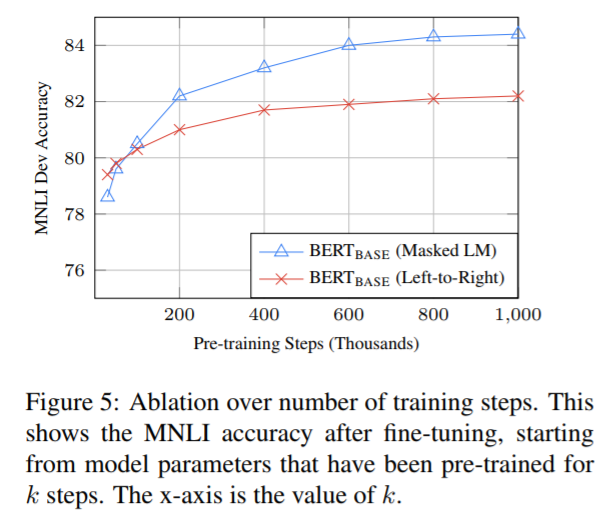In this figure, you can answer the following question:

BERT really need such a huge pre-trained on the order of it (128,000 words / batch * 1000,000 steps)?
Yes. With respect to steps 500k, accuracy can be improved 1.0%

MLM training convergence rate than the pre-LTR slowly? Because each batch, only 15% of the words being predicted, and not all the words are involved.
Do a little bit slow. But accuracy thus immediately over the LTR model, it is worth it.

I said before, the purpose mask strategy is to reduce the mismatch between pre-training and fine-tuning, because [MASK] symbol hardly occur when fine-tuning. Table8 shows the impact of different strategies on the results of MASK-based Fine-tune Feature-based and based on the way:

It can be seen under Feature-based manner, resulting in a greater impact MASK does not match, because the model in training time, feature extraction layer no opportunity to adjust the feature representation (because they were frozen).

In the feature-based method, the output of the last four layers of the BERT stitching together as a feature, such as the best, particularly in Section 5.3.

In addition, we can also see, fine-tuning methods are amazingly robust strategies in different mask. However, as the authors expected, the full use of policy to NER MASK field is problematic in the feature-based way. Interestingly, all use random strategies more than the difference between the strategies of the first row.

annotation

Bidirectional Depth: shallow depth and bidirectional bidirectional difference is that the latter is only trained left-to-right and characterization of the right-to-left separate simple series, and the former is obtained with the training.

feature-based: also known as feature-extraction feature extraction. Is extracted with a pre-trained network on a new sample of the relevant features, these features would then enter a new classifier training process from the beginning. That is to say in the training process, feature extraction layer of the network is to be frozen, and only intensive Link Categories section behind can participate in training.

fine-tuning: fine-tuning. And feature-based difference is that a good training new classifier, they still thawing layers on top of feature extraction layer, and then the classifier joint training again. It is called fine-tuning, because the parameters are updated training on pre-trained parameters, a relatively small change for the better argument than the pre-training, this means that with respect to the relative does not use a pre-trained model parameters to initialize the model downstream tasks an argument. There is also a case, if you have a large number of data samples can be trained, then you can unfreeze all feature extraction layer, all of the parameters involved in the training, but because it is based on the model parameters pre-trained, so there is still training than random initialization way all of the parameters to be faster. BERT for the team of authors use the model in the fine-tuning downstream tasks, on the use of thaw all layers, fine-tuning all parameters of the method.

warmup: learning rate to warm up. In the warm-up before the specified number of steps taken in the process of gradually increasing the learning rate. After the warm-up steps will learn the use of decay rate policy. Such training can avoid the initial shock, so that the latter can drop smaller loss.

ok, Benpian friends – so much content, thanks for reading O (∩_∩) O.